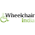## Tuesday, April 3, 2012

### The Travelling salesman problem

The travelling salesman problem is possibly the most famous and probably the most easy to understand of the so-called "NP-hard" problems computer scientists (and mathematicians) have found.

The problem statement is quite simple.

Consider you are a salesman, and you have, say, an $n$ number of cities you've got to cover by the end of the day. But, it would be best if you could minimize the distance that you have to travel between all of the cities, and, you know the coordinates of all the cities in reference to some point known as the *origin*. Given these coordinates, define an algorithm that would give you shortest possible path between the cities.

Of course, this doesn't seem like much of a problem at all when you first start at it (like most other difficult problems); you could just try all the routes between the cities, find the route with the minimum distance covered, and, you're done!

Let's see what that entails in terms of performance/scaling. With $n=5$ (i.e. five cities to visit), we have a total of $5! = 5 \cdot 4 \cdot 3 \cdot 2 \cdot 1 = 120$ ways we can trace out the route between the cities, and we have to check all of these. That doesn't seem too bad for a computer.

How about for $n=10$? Well, $10! = 3,628,800$, so, its quite a big jump from $120$, but, still manageable.

Let's go up by another five; $n=15$. That gives: $15! = 1,307,674,368,000$. Holy cow. That's a lot. But, how did it jump that quickly?!

To answer that question, we'll need a bit of math (if you understand big Oh notation, you can skip the next couple of paragraphs).

Consider this situation. You have one car that's accelerating from a low speed, whereas another car is going at a higher speed, but, the second car's speed will not change. What do you think will happen if we let the cars go on forever? Of course, the car that's accelerating will overtake the car that's at a constant speed, right?

That's what scaling algorithms is all about. You might have one algorithm that performs better at lowever "sizes" of the input, but, a second algorithm may overtake it if it can "scale better" (i.e. accelerate). So, when we say that an algorithm is $O(n)$, what we mean is that the algorithm performs *linearly*, i.e. for each unit increase in $n$, we get a certain unit increase in the time spent (or, whatever else you're measuring). Similarly, $O(n^2)$ means that for every unit increase in $n$, we get that much of change, but, squared in output.

But, all of this still seems on some shaky ground, so, let's break out the equations (warning: if you don't know how limits work, skip this). This won't be a *completely* rigorous argument (which would require more math), but, it will be much better than the argument we have in place.

How can we say that a linear time algorithm (i.e. $O(n)$) will scale better than $O(n^2)$? Well, what we're informally saying is that the $O(n^2)$ grows much quicker (i.e. worse) than $O(n)$ as $n \to +\infty$. If you remember that bit about relative rates of growth from first semester calculus; $\lim_{n \to +\infty} \frac{n^2}{n} = \lim_{n \to +\infty} n = +\infty$

So, we've proved that $O(n^2)$ grows faster than $O(n)$, and therefore performs worse than $O(n)$.

What does that mean in terms of the traveling salesman problem? The traveling salesman problem brute force solution is in fact $O(n!)$ (since the number of routes goes up like that).

Why is this so bad?

Consider a polynomial time solution (i.e. $O(a_n x^n + a_{n-1} x^{n-1} ... + a_o)$). Using a bit of calculus/limits, we can say, for certain, that factorial algorithms are much worse than polynomial time solutions as $n \to \infty$.

That's what makes the travelling salesman problem interesting, because it has a non-polynomial solution, and, its something called a NP-Hard problem. What NP-Hard means is actually very complicated and heavily theoretical, but, it consitutes a very important part of the P vs NP problem, which, informally, asks (quoted from Wikipedia): "whether every problem whose solution can be quickly verified by a computer can also be quickly solved by a computer" (s/quickly/polynomial time/g).

So, that's what the travelling salesman problem is, and why people care.

1.2.